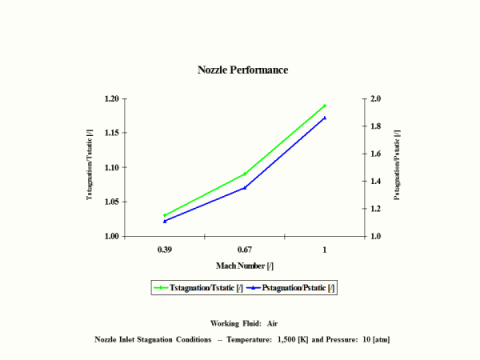top of page

### Nozzle Analysis

Nozzle

Introduction

This section provides an isentropic nozzle analysis when the working fluid is air.

Analysis

In the presented nozzle analysis, only air is considered as the working fluid behaving as a perfect gas -- specific heat has a constant value.  Ideal gas state equation is valid -- pv = RT.

Air enters a nozzle at point 1 and it exits the nozzle at point 2.  Isentropic expansion is considered with no entropy change.

Figure 1 contains a nozzle schematic layout.Figure 1 - Nozzle Schematic Layout

Figure 2 presents a nozzle temperature vs entropy diagram.Figure 2 - Nozzle Temperature vs Entropy Diagram

Figure 3 presents nozzle performance -- stagnation over static temperature and pressure ratio values as a function of the Mach Number.  Only subsonic nozzle operation is considered.  It should be noted that air enters the nozzle at the stagnation conditions of 1,500 [K] and 10 [atm] of absolute pressure.Figure 3 - Nozzle Performance

One can notice that nozzle stagnation over static temperature and pressure ratio values increase with an increase in the Mach Number.

Assumptions

Working fluid is air.  There is no friction and heat transfer.  Expansion is isentropic -- there is no entropy change.  Ideal gas state equation is valid -- pv = RT.  Air behaves as a perfect gas -- specific heat has a constant value.

Governing Equations

Tt/T = (1 + M^2(k - 1)/2)
p
t/p = (1 + M^2(k - 1)/2)^
ϰ/(ϰ-1)
T
t/T = (pt/p)^(
ϰ-1)/ϰ
v = (2c
p(Tt - T))1/2
v
s = (
ϰRT)^1/2

M = v/vs

ϰ = cp/cv
c
p - cv = R
pv = RT

Input Data

T1 = 1,500 [K]
p
1 = 10 [atm]
R = 0.2867 [kJ/kg*K]
c
p = 1.004 [kJ/kg*K]

ϰ = 1.4 [/]

M = 0.39, 0.67 and 1 [/]

Results

Nozzle Performance vs Outlet Mach Number
Nozzle Inlet Stagnation Temperature = 1,500 [K] and Pressure = 10 [atm]

Outlet Mach
Number

[/]

0.39

0.67

1.00

Stagnation/Static
Temperature Ratio

[/]

1.03

1.09

1.19

Stagnation/Static
Pressure Ratio

[/]

1.11

1.35

1.86

Conclusions

Nozzle stagnation over static temperature and pressure ratio values increase with an increase in the Mach Number.

References

JANAF Thermochemical Data - Tables, 1970

bottom of page